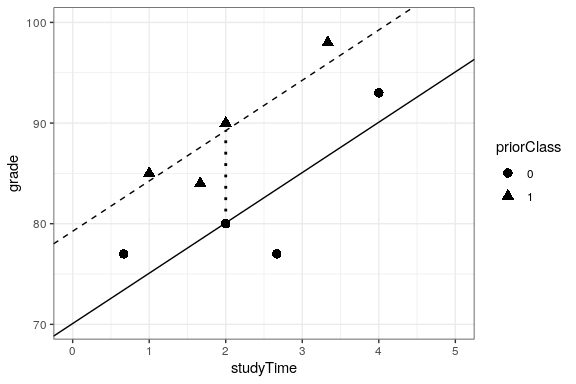# 26.2: Fitting More Complex Models

Often we would like to understand the effects of multiple variables on some particular outcome, and how they relate to one another. In the context of our study time example, let’s say that we discovered that some of the students had previously taken a course on the topic. If we plot their grades (see Figure 26.3), we can see that those who had a prior course perform much better than those who had not, given the same amount of study time. We would like to build a statistical model that takes this into account, which we can do by extending the model that we built above:

$\hat{y} = \hat{\beta_1}*studyTime + \hat{\beta_2}*priorClass + \hat{\beta_0}$ To model whether each individual has had a previous class or not, we use what we call dummy coding in which we create a new variable that has a value of one to represent having had a class before, and zero otherwise. This means that for people who have had the class before, we will simply add the value of $\hat{\beta_2}$ to our predicted value for them – that is, using dummy coding $\hat{\beta_2}$ simply reflects the difference in means between the two groups. Our estimate of $\hat{\beta_1}$ reflects the regression slope over all of the data points – we are assuming that regression slope is the same regardless of whether someone has had a class before (see Figure 26.3).

##
## Call:
## lm(formula = grade ~ studyTime + priorClass, data = df)
##
## Residuals:
##       1       2       3       4       5       6       7       8
##  3.5833  0.7500 -3.5833 -0.0833  0.7500 -6.4167  2.0833  2.9167
##
## Coefficients:
##             Estimate Std. Error t value Pr(>|t|)
## (Intercept)    70.08       3.77   18.60  8.3e-06 ***
## studyTime       5.00       1.37    3.66    0.015 *
## priorClass1     9.17       2.88    3.18    0.024 *
## ---
## Signif. codes:  0 '***' 0.001 '**' 0.01 '*' 0.05 '.' 0.1 ' ' 1
##
## Residual standard error: 4 on 5 degrees of freedom
## Multiple R-squared:  0.803,  Adjusted R-squared:  0.724
## F-statistic: 10.2 on 2 and 5 DF,  p-value: 0.0173Figure 26.3: The relation between study time and grade including prior experience as an additional component in the model. The solid line relates study time to grades for students who have not had prior experience, and the dashed line relates grades to study time for students with prior experience. The dotted line corresponds to the difference in means between the two groups.